Test: Matrices & Determinants - 2

# Test: Matrices & Determinants - 2

Test Description

## 20 Questions MCQ Test IIT JAM Mathematics | Test: Matrices & Determinants - 2

Test: Matrices & Determinants - 2 for Mathematics 2023 is part of IIT JAM Mathematics preparation. The Test: Matrices & Determinants - 2 questions and answers have been prepared according to the Mathematics exam syllabus.The Test: Matrices & Determinants - 2 MCQs are made for Mathematics 2023 Exam. Find important definitions, questions, notes, meanings, examples, exercises, MCQs and online tests for Test: Matrices & Determinants - 2 below.
Solutions of Test: Matrices & Determinants - 2 questions in English are available as part of our IIT JAM Mathematics for Mathematics & Test: Matrices & Determinants - 2 solutions in Hindi for IIT JAM Mathematics course. Download more important topics, notes, lectures and mock test series for Mathematics Exam by signing up for free. Attempt Test: Matrices & Determinants - 2 | 20 questions in 20 minutes | Mock test for Mathematics preparation | Free important questions MCQ to study IIT JAM Mathematics for Mathematics Exam | Download free PDF with solutions
 1 Crore+ students have signed up on EduRev. Have you?
Test: Matrices & Determinants - 2 - Question 1

### The inverse of the matrix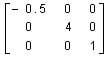is

Test: Matrices & Determinants - 2 - Question 2

### If the rank of a matrix A is 2, then the rank of 2A is

Test: Matrices & Determinants - 2 - Question 3

### If A =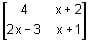is symmetric, then x =

Test: Matrices & Determinants - 2 - Question 4

The matrix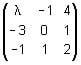is invertible if

Test: Matrices & Determinants - 2 - Question 5

If A is a skew-symmetric matrix, then trace of A is

Test: Matrices & Determinants - 2 - Question 6

If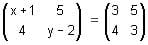then x and y will be

Test: Matrices & Determinants - 2 - Question 7

Matrix has a value. This statement

Test: Matrices & Determinants - 2 - Question 8

If  then X is equal to

Test: Matrices & Determinants - 2 - Question 9

If A =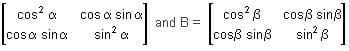are two matrices such that the product AB is a null matrix, then -is

Test: Matrices & Determinants - 2 - Question 10

If A is a square matrix such that AA` = I, then value of A`A is

Test: Matrices & Determinants - 2 - Question 11

If A is a square matrix of order n such that its elements are polynomial in x and its r-rows become identical for x = k, then

Test: Matrices & Determinants - 2 - Question 12

If A is a 3-rowed square matrix, then | 5 A | is equal to

Test: Matrices & Determinants - 2 - Question 13

In the determinant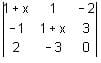, the ratio of the cofactor to its minor of the element – 3 is

Test: Matrices & Determinants - 2 - Question 14

The solution set of the equation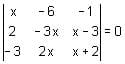is

Test: Matrices & Determinants - 2 - Question 15

If ω is an imaginary cube root of unity and x is real number, then which one of the following is a factor of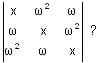Test: Matrices & Determinants - 2 - Question 16

If A is a square matrix of order 4, and I is a unit matrix, then it is true that

Test: Matrices & Determinants - 2 - Question 17

If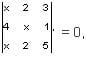then x is

Test: Matrices & Determinants - 2 - Question 18

The roots of the equation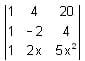= 0 are

Test: Matrices & Determinants - 2 - Question 19

The value of determinant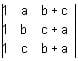is

Test: Matrices & Determinants - 2 - Question 20

The value of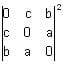## IIT JAM Mathematics

106 videos|27 docs|30 tests
Information about Test: Matrices & Determinants - 2 Page
In this test you can find the Exam questions for Test: Matrices & Determinants - 2 solved & explained in the simplest way possible. Besides giving Questions and answers for Test: Matrices & Determinants - 2, EduRev gives you an ample number of Online tests for practice

## IIT JAM Mathematics

106 videos|27 docs|30 tests(Scan QR code)